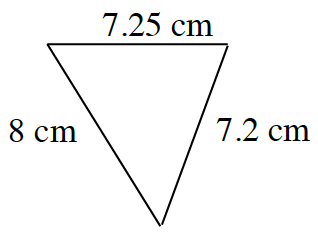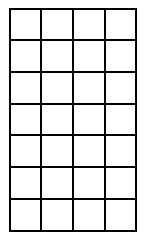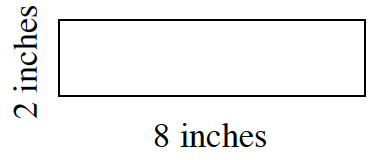Home > CC1MN > Chapter cc13 > Lesson cc13.1.1 > Problem3-15

3-15.

Find the measure indicated in each question below. Homework Help ✎

 Find the perimeter of the triangle.Hint (a):Remember that perimeter is the sum of the border lengths of the shape.b. Find the area of the large rectangle.Hint (b):Remember that area is the number of square units in a shape.
1. Find the area and perimeter of the rectangle at right.

See parts (a) and (b).$\text{Area} = 16 \text{ sq. inches}$
$\text{Perimeter} = 20 \text{ inches}$
Be sure to know how to find these answers using the given measurements.